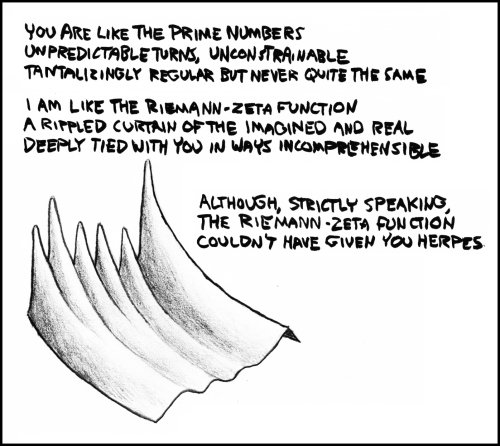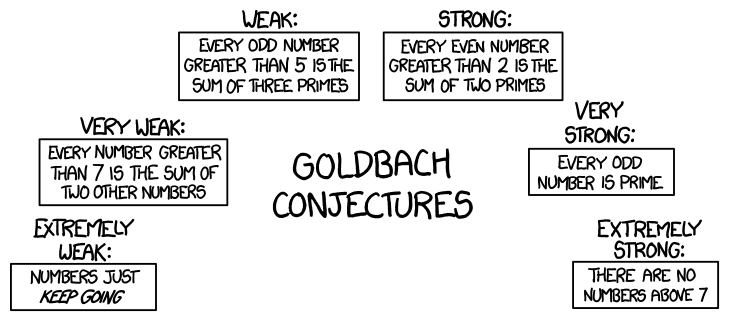MoscaJoined: Dec 14, 2009
• Posts: 3815
January 3rd, 2014 at 12:01:19 PM permalink
I was searching for something else and found this, I figure some folks would like it. Funny enough, I know what the Riemann-Zeta function is. Not the math, of course.NO KILL I
MoscaJoined: Dec 14, 2009
• Posts: 3815
January 3rd, 2014 at 12:13:57 PM permalink
This was what I was looking for. I sort of get it, but could use a better explanation if someone feels like giving me one.The weak twin primes conjecture states that there are infinitely many pairs of primes. The strong twin primes conjecture states that every prime p has a twin prime (p+2), although (p+2) may not look prime at first. The tautological prime conjecture states that the tautological prime conjecture is true.
NO KILL I
AxiomOfChoiceJoined: Sep 12, 2012
• Posts: 5761
January 3rd, 2014 at 1:24:25 PM permalink
What are you asking? To explain the joke?

IMO it's not that funny, but the two statements on the left are obviously true, and the two statements on the right are obviously false. The "Strong" and "Weak" are open problems (the Goldbach Conjecture) with "Strong" being a stronger statement than "Weak" (ie, Strong ==> Weak).

The joke is that there are several statements collectively known as "strong" or "weak" versions of the goldbach conjecture. When people say "The Goldbach Conjecture" they usually mean the one that is labelled "Strong" in the comic.

http://en.wikipedia.org/wiki/Goldbach_conjecture might help.
MoscaJoined: Dec 14, 2009
• Posts: 3815
January 3rd, 2014 at 2:30:01 PM permalink
Allowing for differences in humor, XKCD usually is at least worth a chuckle. There has to be some joke in the fact that the weaker the conjecture, the more obviously true, and the stronger the conjecture, the more obviously false.

edit: I found it.

Quote:

Randall is riffing on the relationship between "strong" and "weak" logical statements, which are an interplay between the boldness or usefulness of a statement and the ease with which it might be proven to be true. For example, if Goldbach's conjecture (given in the comic under the label "strong") could be proven to be true, it would automatically imply that Goldbach's weak conjecture (given in the comic under the label "weak") is also true, because any odd number greater than 5 can be expressed as 3 (a prime number) plus an even number greater than 2 (which, per the strong conjecture, would itself be the sum of two prime numbers), resulting in a way to express the original odd number as the sum of three prime numbers. The weak conjecture does not, however, imply the strong conjecture.

Mathematicians have been solving related problems that are "weaker" than the weak conjecture, and working towards "stronger" ones. For example, in 1937 the weak conjecture was proven for odd numbers greater than 314348907. In 1995 a version was proven based on the sum of no more than seven prime numbers, and in 2012 the ceiling was lowered to five primes. In 2013 the weak conjecture was claimed proven for numbers greater than 1030, while all numbers below 1030 have been verified by supercomputer to satisfy the conjecture; these together imply that the weak conjecture is true (although there is no general proof of it for all numbers). Goldbach's strong conjecture remains unsolved.

This comic plays on the "strong" and "weak" naming of Goldbach's conjectures by extending it to further degrees of strength or weakness. The "very weak" and "extremely weak" conjectures are indeed implied by Goldbach's weak conjecture, just as the weak conjecture is implied by the strong one. The "very strong" and "extremely strong" conjectures are extensions of Goldbach's strong conjecture, even as it is an extension of the weak conjecture. However, the "very weak" and "extremely weak" conjectures are so obviously true that they are hardly worth stating, while the "very strong" and "extremely strong" conjectures make such bold claims that they are obviously false.

Moreover, the "extremely weak" and "extremely strong" conjectures contradict each other, even though they're both derived (albeit in opposite directions) from the same initial conjectures.

http://www.explainxkcd.com/wiki/index.php/1310:_Goldbach_Conjectures
NO KILL I
98ClubsJoined: Jun 3, 2010
• Posts: 1728
January 3rd, 2014 at 3:34:55 PM permalink
ONE is NOT a prime, its "UNIQUE" in that its divisible by one and itself only, but one IS itself. TWO is the ONLY even prime discovered. All primes greater than 6 have a terminal digit of either 1, 3, 7, or 9. (2 and 5 are the only exceptions known otherwise it would be all primes)
Some people need to reimagine their thinking.
AxiomOfChoiceJoined: Sep 12, 2012
• Posts: 5761
January 3rd, 2014 at 3:39:59 PM permalink
Quote: 98Clubs

TWO is the ONLY even prime discovered.

Do you suspect that there are undiscovered ones?
98ClubsJoined: Jun 3, 2010
• Posts: 1728
January 3rd, 2014 at 3:56:03 PM permalink
No. Like my statement about "one" thats how I learned... so far its holding up. ;o)
Some people need to reimagine their thinking.
AxiomOfChoiceJoined: Sep 12, 2012